Most Affordable JEE | NEET | 8,9,10 Preparation by Kota's Top IITian Doctor Faculties

# NCERT Solutions for Class 9 Maths chapter 8 Exercise 8.2 - Quadrilaterals

Class 9Hey, are you a class 9 Student and Looking for Ways to Download NCERT Solutions for Class 9 Maths chapter 8 Exercise 8.2? If Yes then you are at the right place.

Here we have listed Class 9 maths chapter 8 exercise 8.2 solutions in PDF that is prepared by Kota’s top IITian’s Faculties by keeping Simplicity in mind.

If you want to score high in your class 9 Maths Exam then it is very important for you to have a good knowledge of all the important topics, so to learn and practice those topics you can use eSaral NCERT Solutions.

So, without wasting more time Let’s start.

### Download The PDF of NCERT Solutions for Class 9 Maths chapter 8 Exercise 8.2 "Quadrilaterals"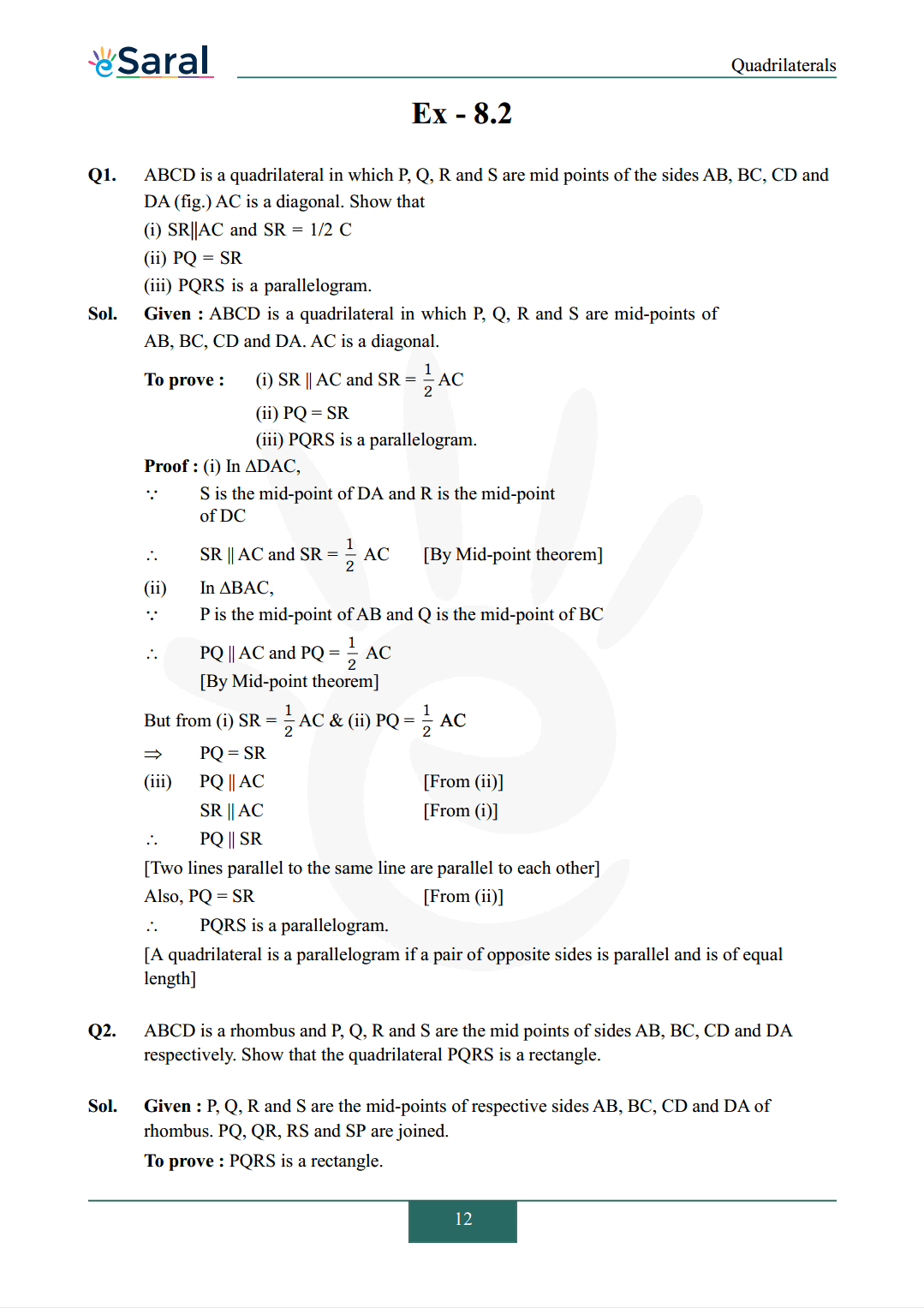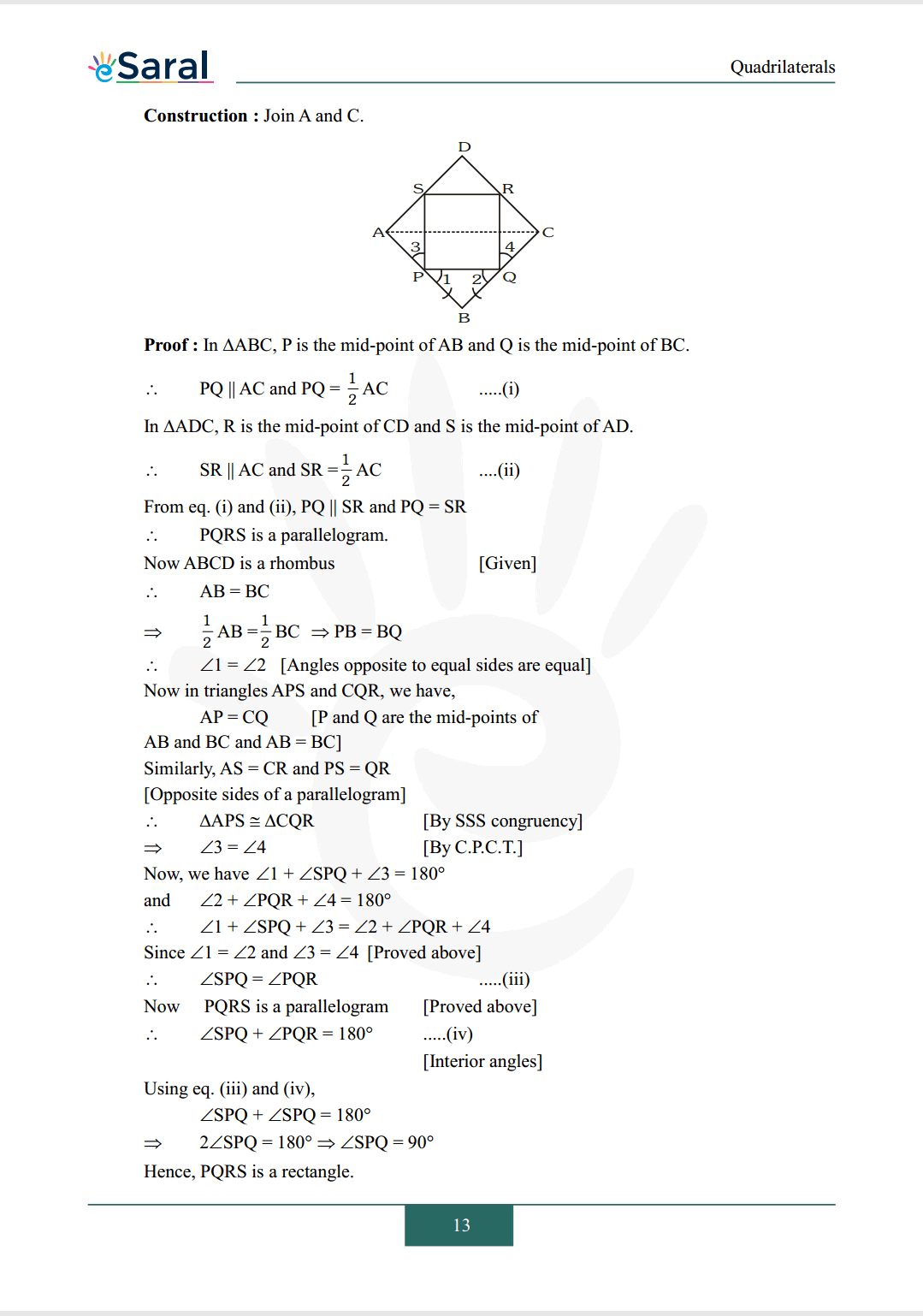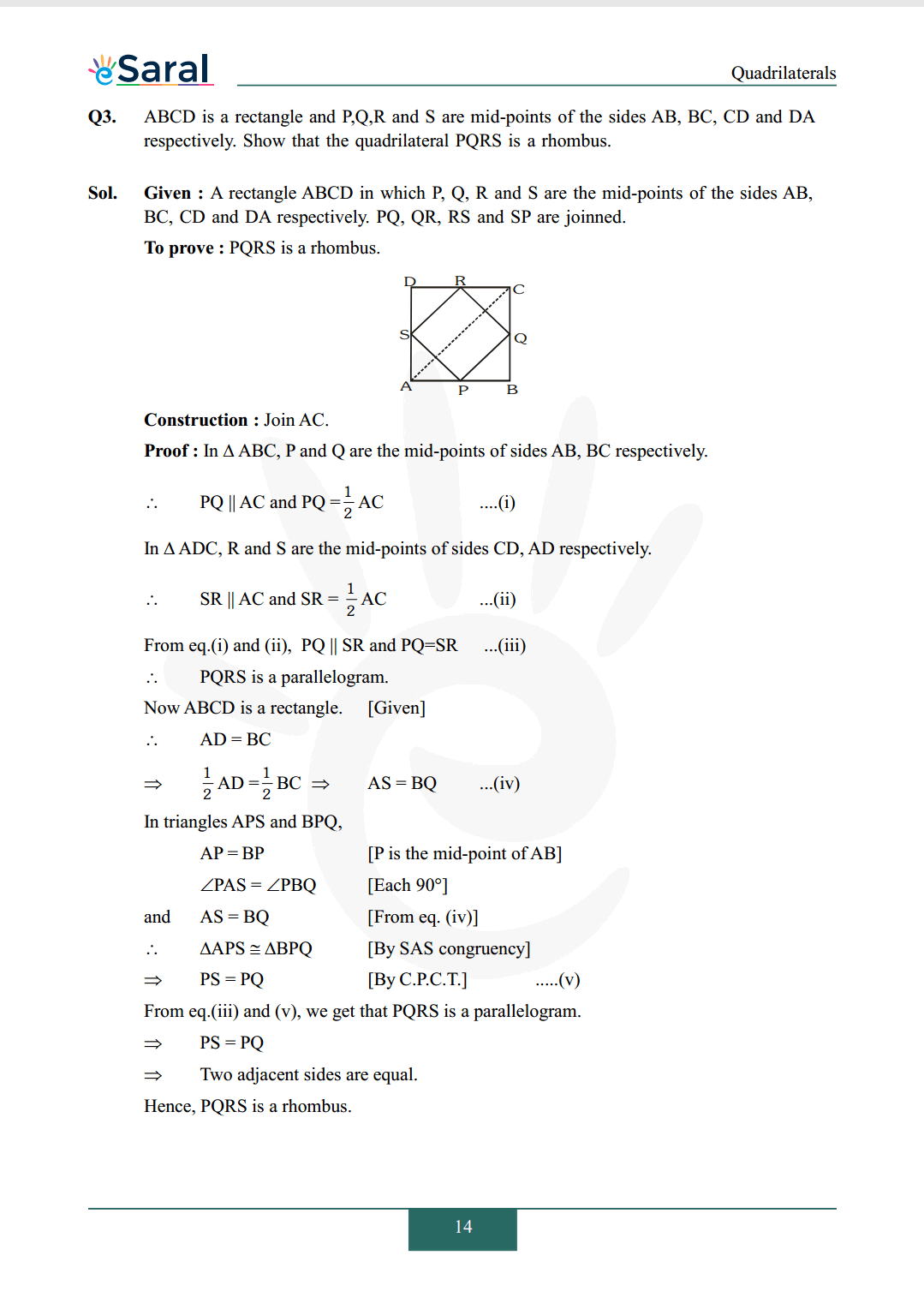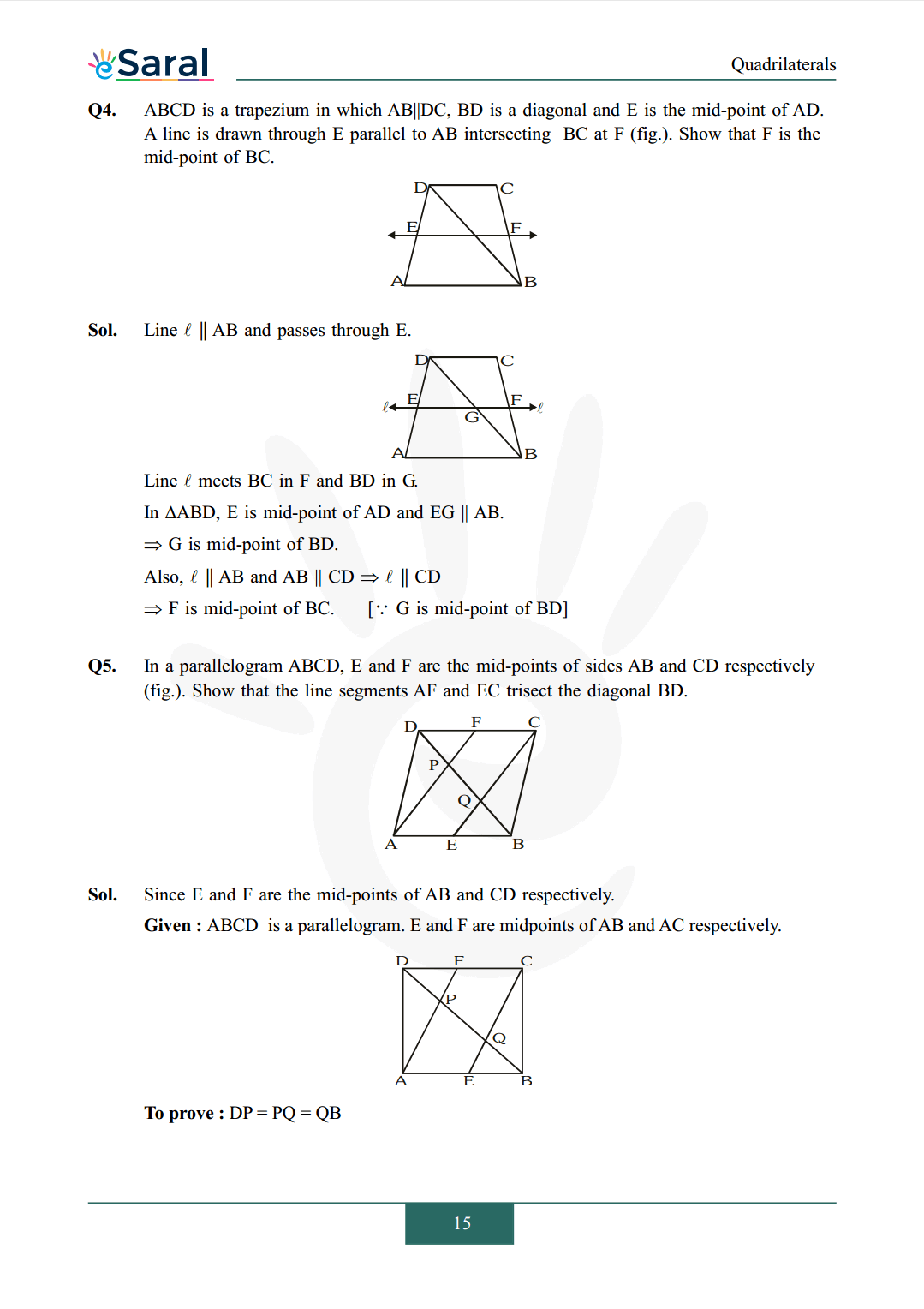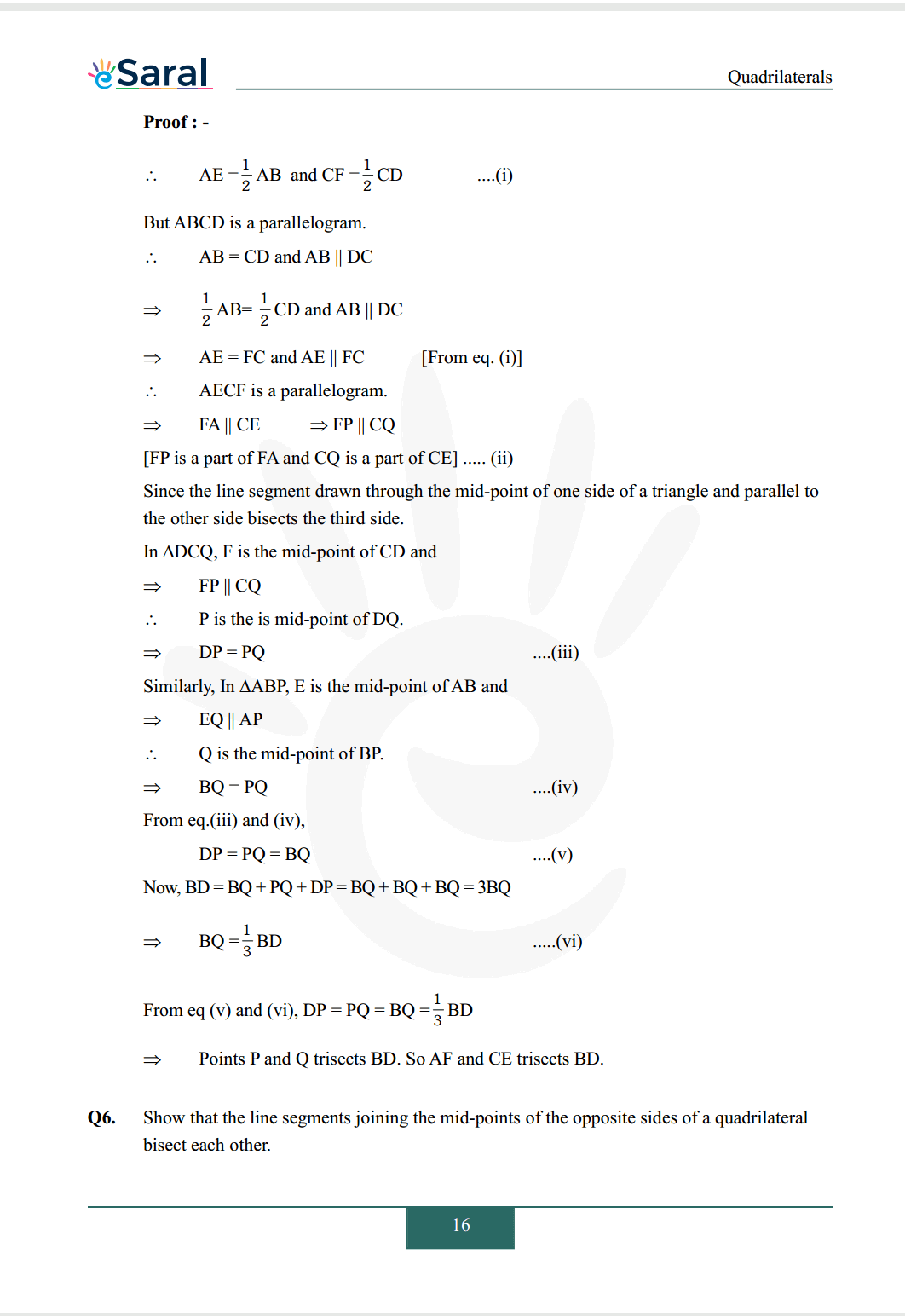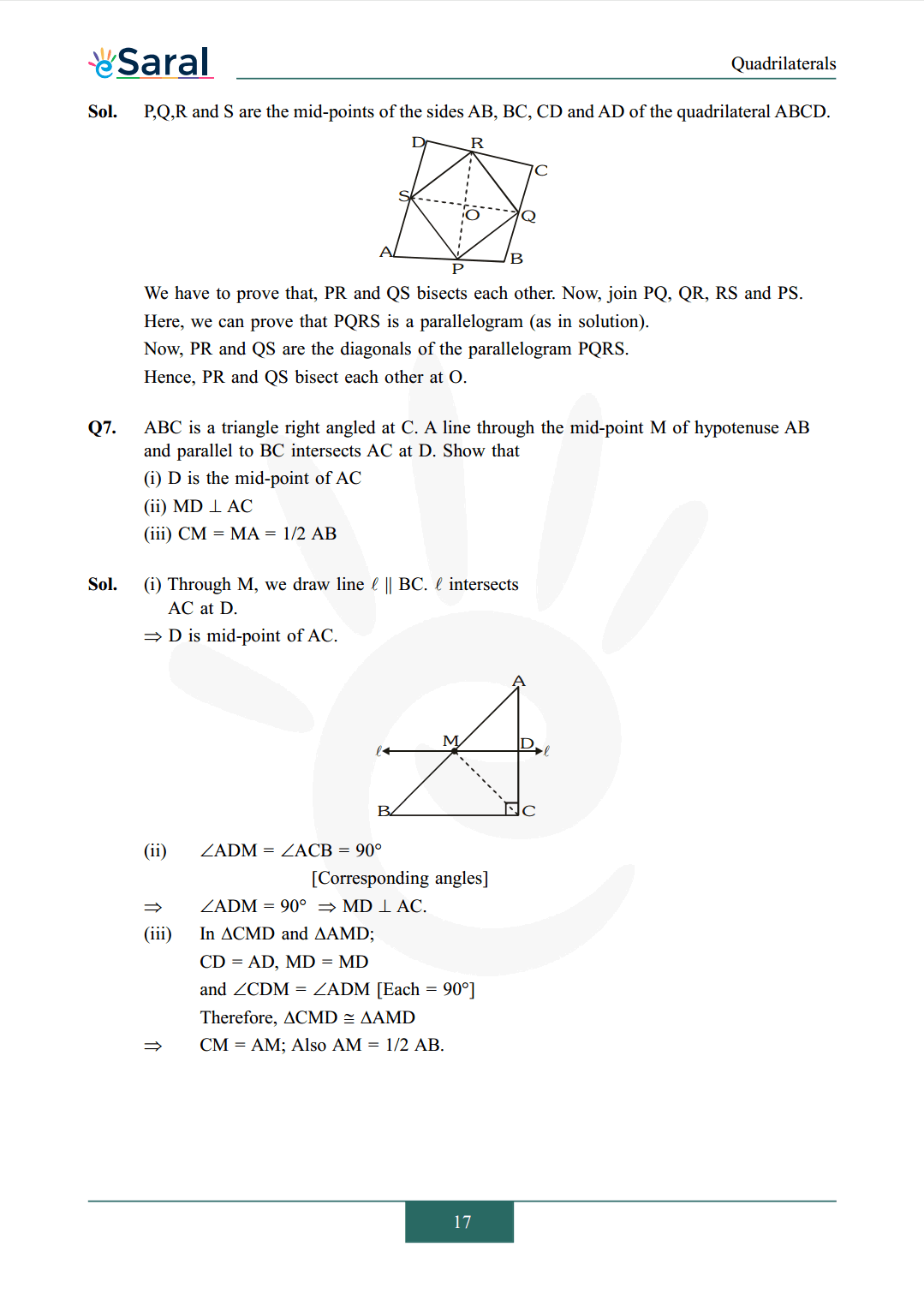#### All Questions of Chapter 8 Exercise 8.2

Once you complete the chapter 8 then you can revise Ex. 8.2 by solving following questions

Q1. $\quad \mathrm{ABCD}$ is a quadrilateral in which $\mathrm{P}, \mathrm{Q}, \mathrm{R}$ and $\mathrm{S}$ are mid points of the sides $\mathrm{AB}, \mathrm{BC}, \mathrm{CD}$ and DA (fig.) $\mathrm{AC}$ is a diagonal. Show that
(i) $\mathrm{SR} \| \mathrm{AC}$ and $\mathrm{SR}=1 / 2 \mathrm{C}$
(ii) $\mathrm{PQ}=\mathrm{SR}$
(iii) PQRS is a parallelogram.

Q2. $\mathrm{ABCD}$ is a rhombus and $\mathrm{P}, \mathrm{Q}, \mathrm{R}$ and $\mathrm{S}$ are the mid points of sides $\mathrm{AB}, \mathrm{BC}, \mathrm{CD}$ and $\mathrm{DA}$ respectively. Show that the quadrilateral PQRS is a rectangle.

Q3. $\mathrm{ABCD}$ is a rectangle and $\mathrm{P}, \mathrm{Q}, \mathrm{R}$ and $\mathrm{S}$ are mid-points of the sides $\mathrm{AB}, \mathrm{BC}, \mathrm{CD}$ and $\mathrm{DA}$ respectively. Show that the quadrilateral PQRS is a rhombus.

Q4. $\mathrm{ABCD}$ is a trapezium in which $\mathrm{AB} \| \mathrm{DC}, \mathrm{BD}$ is a diagonal and $\mathrm{E}$ is the mid-point of $\mathrm{AD}$. A line is drawn through $\mathrm{E}$ parallel to $\mathrm{AB}$ intersecting $\mathrm{BC}$ at $\mathrm{F}$ (fig.). Show that $\mathrm{F}$ is the mid-point of $\mathrm{BC}$.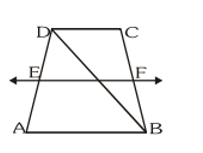Q5. In a parallelogram $\mathrm{ABCD}, \mathrm{E}$ and $\mathrm{F}$ are the mid-points of sides $\mathrm{AB}$ and $\mathrm{CD}$ respectively (fig.). Show that the line segments $\mathrm{AF}$ and EC trisect the diagonal $\mathrm{BD}$.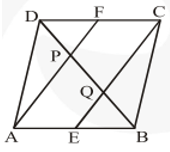Q6. Show that the line segments joining the mid-points of the opposite sides of a quadrilateral bisect each other.

Q7. $\mathrm{ABC}$ is a triangle right angled at $\mathrm{C}$. A line through the mid-point $\mathrm{M}$ of hypotenuse $\mathrm{AB}$ and parallel to BC intersects $\mathrm{AC}$ at $\mathrm{D}$. Show that
(i) $\mathrm{D}$ is the mid-point of $\mathrm{AC}$
(ii) $\mathrm{MD} \perp \mathrm{AC}$
(iii) $\mathrm{CM}=\mathrm{MA}=1 / 2 \mathrm{AB}$